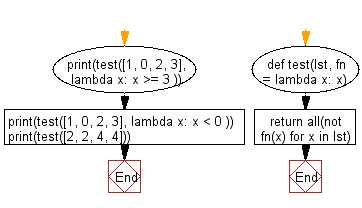﻿ Python: Check if a given function returns True for at least one element in the list - w3resource# Python: Check if a given function returns True for at least one element in the list

## Python List: Exercise - 259 with Solution

Write a Python program to check if a given function returns True for at least one element in the list.

Use all() and fn to check if fn returns False for all the elements in the list.

Sample Solution:

Python Code:

``````def test(lst, fn = lambda x: x):
return all(not fn(x) for x in lst)
print(test([1, 0, 2, 3], lambda x: x >= 3 ))
print(test([1, 0, 2, 3], lambda x: x < 0 ))
print(test([2, 2, 4, 4]))
```
```

Sample Output:

```False
True
False
```

Flowchart:## Visualize Python code execution:

The following tool visualize what the computer is doing step-by-step as it executes the said program:

Python Code Editor:

Have another way to solve this solution? Contribute your code (and comments) through Disqus.

What is the difficulty level of this exercise?

Test your Python skills with w3resource's quiz

﻿

## Python: Tips of the Day

```print(2_000_000)
```2000000